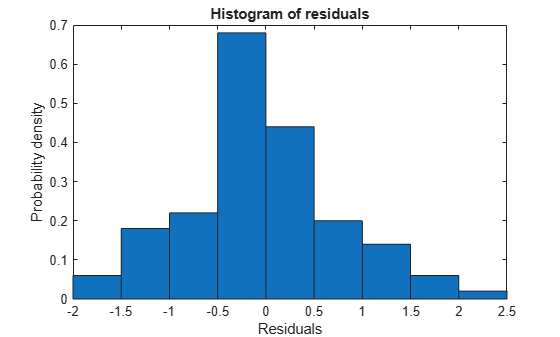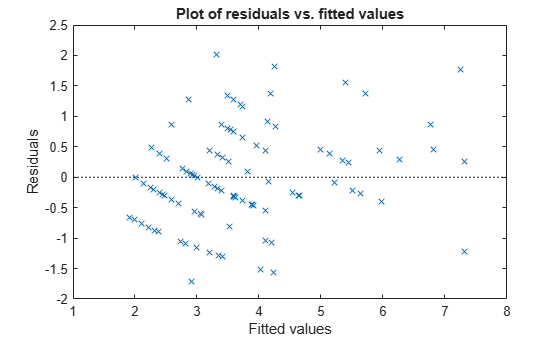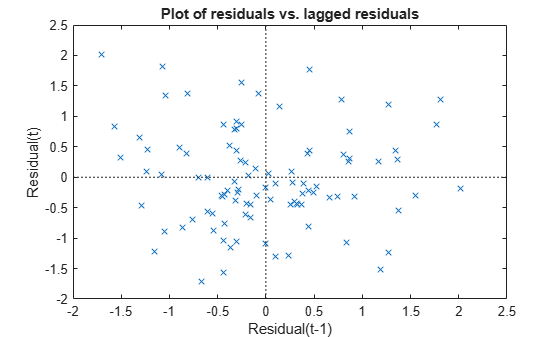# plotResiduals

Plot residuals of generalized linear mixed-effects model

## Syntax

``plotResiduals(glme,plottype)``
``plotResiduals(glme,plottype,Name,Value)``
``h = plotResiduals(___)``

## Description

````plotResiduals(glme,plottype)` plots the raw conditional residuals of the generalized linear mixed-effects model `glme` in a plot of the type specified by `plottype`.```

example

````plotResiduals(glme,plottype,Name,Value)` plots the conditional residuals of `glme` using additional options specified by one or more `Name,Value` pair arguments. For example, you can specify to plot the Pearson residuals.```
````h = plotResiduals(___)` returns a handle, `h`, to the lines or patches in the plot of residuals.```

## Input Arguments

expand all

Generalized linear mixed-effects model, specified as a `GeneralizedLinearMixedModel` object. For properties and methods of this object, see `GeneralizedLinearMixedModel`.

Type of residual plot, specified as one of the following.

ValueDescription
`'histogram'`Histogram of residuals
`'caseorder'`Residuals versus case order. Case order is the same as the row order used in the input data `tbl` when fitting the model using `fitglme`.
`'fitted'`Residuals versus fitted values
`'lagged'`Residuals versus lagged residual (r(t) versus r(t – 1))
`'probability'`Normal probability plot
`'symmetry'` Symmetry plot

Example: `plotResiduals(glme,'lagged')`

### Name-Value Pair Arguments

Specify optional comma-separated pairs of `Name,Value` arguments. `Name` is the argument name and `Value` is the corresponding value. `Name` must appear inside quotes. You can specify several name and value pair arguments in any order as `Name1,Value1,...,NameN,ValueN`.

Residual type, specified by the comma-separated pair consisting of `ResidualType` and one of the following.

Residual TypeFormula
`'raw'`

`${r}_{ci}={y}_{i}-{g}^{-1}\left({x}_{i}^{T}\stackrel{^}{\beta }+{z}_{i}^{T}\stackrel{^}{b}+{\delta }_{i}\right)$`

`'Pearson'`

`${r}_{ci}^{pearson}=\frac{{r}_{ci}}{\sqrt{\frac{\stackrel{^}{{\sigma }^{2}}}{{w}_{i}}{v}_{i}\left({\mu }_{i}\left(\stackrel{^}{\beta },\stackrel{^}{b}\right)\right)}}$`

In each of these equations:

• yi is the ith element of the n-by-1 response vector, y, where i = 1, ..., n.

• g-1 is the inverse link function for the model.

• xiT is the ith row of the fixed-effects design matrix X.

• ziT is the ith row of the random-effects design matrix Z.

• δi is the ith offset value.

• σ2 is the dispersion parameter.

• wi is the ith observation weight.

• vi is the variance term for the ith observation.

• μi is the mean of the response for the ith observation.

• $\stackrel{^}{\beta }$ and $\stackrel{^}{b}$ are estimated values of β and b.

Raw residuals from a generalized linear mixed-effects model have nonconstant variance. Pearson residuals are expected to have an approximately constant variance, and are generally used for analysis.

Example: `'ResidualType','Pearson'`

## Output Arguments

expand all

Handle to the residual plot, returned as a graphics object. You can use dot notation to change certain property values of the object, including face color for a histogram, and marker style and color for a scatterplot. For more information, see Access Property Values (MATLAB).

## Examples

expand all

`load mfr`

This simulated data is from a manufacturing company that operates 50 factories across the world, with each factory running a batch process to create a finished product. The company wants to decrease the number of defects in each batch, so it developed a new manufacturing process. To test the effectiveness of the new process, the company selected 20 of its factories at random to participate in an experiment: Ten factories implemented the new process, while the other ten continued to run the old process. In each of the 20 factories, the company ran five batches (for a total of 100 batches) and recorded the following data:

• Flag to indicate whether the batch used the new process (`newprocess`)

• Processing time for each batch, in hours (`time`)

• Temperature of the batch, in degrees Celsius (`temp`)

• Categorical variable indicating the supplier (`A`, `B`, or `C`) of the chemical used in the batch (`supplier`)

• Number of defects in the batch (`defects`)

The data also includes `time_dev` and `temp_dev`, which represent the absolute deviation of time and temperature, respectively, from the process standard of 3 hours at 20 degrees Celsius.

Fit a generalized linear mixed-effects model using `newprocess`, `time_dev`, `temp_dev`, and `supplier` as fixed-effects predictors. Include a random-effects term for intercept grouped by `factory`, to account for quality differences that might exist due to factory-specific variations. The response variable `defects` has a Poisson distribution, and the appropriate link function for this model is log. Use the Laplace fit method to estimate the coefficients. Specify the dummy variable encoding as `'effects'`, so the dummy variable coefficients sum to 0.

The number of defects can be modeled using a Poisson distribution:

`${\text{defects}}_{ij}\sim \text{Poisson}\left({\mu }_{ij}\right)$`

This corresponds to the generalized linear mixed-effects model

`$\mathrm{log}\left({\mu }_{ij}\right)={\beta }_{0}+{\beta }_{1}{\text{newprocess}}_{ij}+{\beta }_{2}{\text{time}\text{_}\text{dev}}_{ij}+{\beta }_{3}{\text{temp}\text{_}\text{dev}}_{ij}+{\beta }_{4}{\text{supplier}\text{_}\text{C}}_{ij}+{\beta }_{5}{\text{supplier}\text{_}\text{B}}_{ij}+{b}_{i},$`

where

• ${\text{defects}}_{ij}$ is the number of defects observed in the batch produced by factory $i$ during batch $j$.

• ${\mu }_{ij}$ is the mean number of defects corresponding to factory $i$ (where $i=1,2,...,20$) during batch $j$ (where $j=1,2,...,5$).

• ${\text{newprocess}}_{ij}$, ${\text{time}\text{_}\text{dev}}_{ij}$, and ${\text{temp}\text{_}\text{dev}}_{ij}$ are the measurements for each variable that correspond to factory $i$ during batch $j$. For example, ${\text{newprocess}}_{ij}$ indicates whether the batch produced by factory $i$ during batch $j$ used the new process.

• ${\text{supplier}\text{_}\text{C}}_{ij}$ and ${\text{supplier}\text{_}\text{B}}_{ij}$ are dummy variables that use effects (sum-to-zero) coding to indicate whether company `C` or `B`, respectively, supplied the process chemicals for the batch produced by factory $i$ during batch $j$.

• ${b}_{i}\sim N\left(0,{\sigma }_{b}^{2}\right)$ is a random-effects intercept for each factory $i$ that accounts for factory-specific variation in quality.

`glme = fitglme(mfr,'defects ~ 1 + newprocess + time_dev + temp_dev + supplier + (1|factory)','Distribution','Poisson','Link','log','FitMethod','Laplace','DummyVarCoding','effects');`

Create diagnostic plots using Pearson residuals to test the model assumptions.

Plot a histogram to visually confirm that the mean of the Pearson residuals is equal to 0. If the model is correct, we expect the Pearson residuals to be centered at 0.

`plotResiduals(glme,'histogram','ResidualType','Pearson')`The histogram shows that the Pearson residuals are centered at 0.

Plot the Pearson residuals versus the fitted values, to check for signs of nonconstant variance among the residuals (heteroscedasticity). We expect the conditional Pearson residuals to have a constant variance. Therefore, a plot of conditional Pearson residuals versus conditional fitted values should not reveal any systematic dependence on the conditional fitted values.

`plotResiduals(glme,'fitted','ResidualType','Pearson')`The plot does not show a systematic dependence on the fitted values, so there are no signs of nonconstant variance among the residuals.

Plot the Pearson residuals versus lagged residuals, to check for correlation among the residuals. The conditional independence assumption in GLME implies that the conditional Pearson residuals are approximately uncorrelated.

`plotResiduals(glme,'lagged','ResidualType','Pearson')`There is no pattern to the plot, so there are no signs of correlation among the residuals.